# backed by data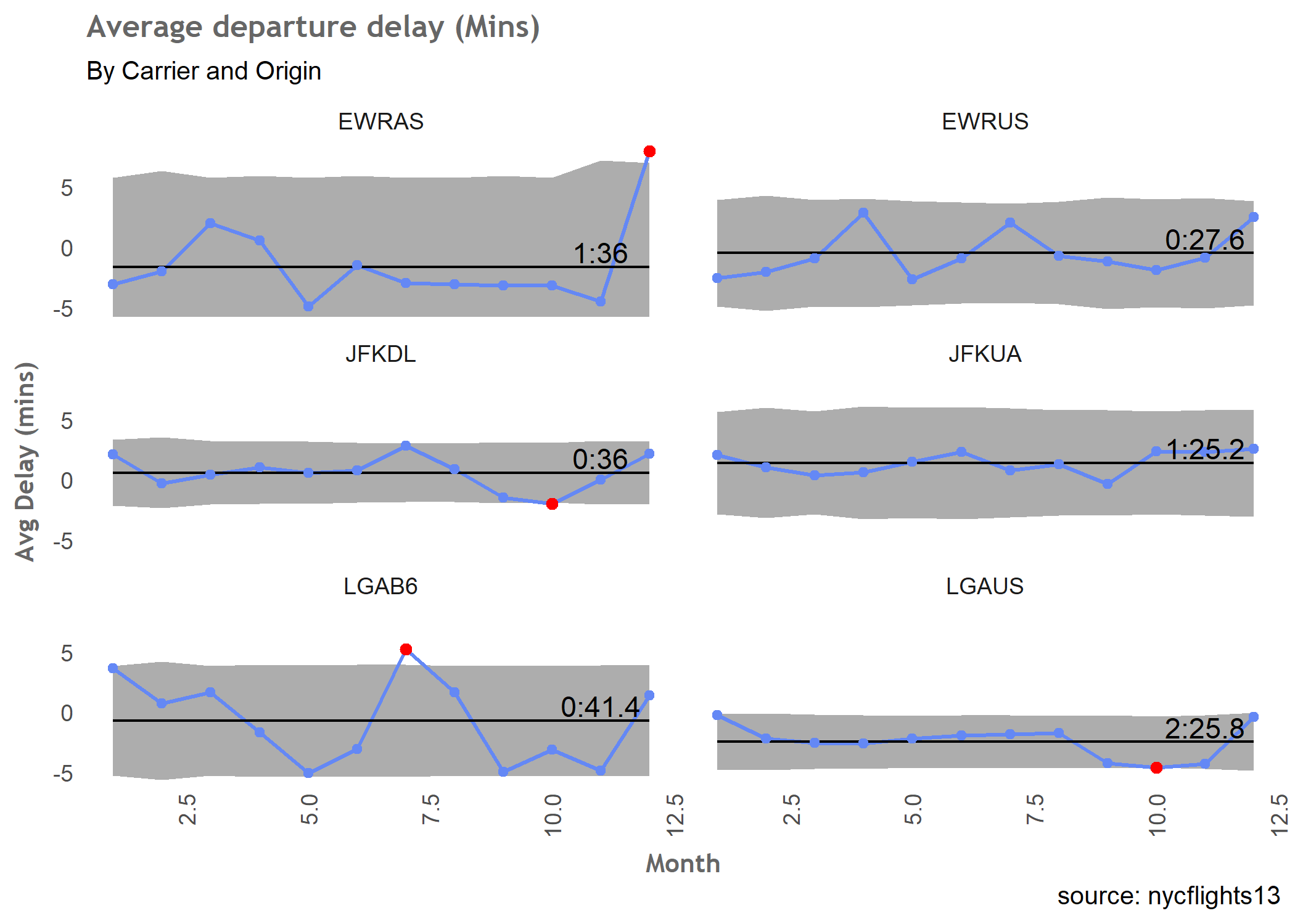Minimising the risk of delayed departure with process control charts

This post is an attempt to answer this question:

It was a pretty interesting question, and I had an initial stab at providing an answer, but the charts I submitted were not the best looking, shall we say ( it was a late night rush job). So, I’m going to hopefully create some nicer looking ones, at least to my poor sleep deprived eyes.

Required libraries - most you will have, but qicharts2 and skimr may be new to you

library(dplyr)
library(scales)
library(ggplot2)
library(nycflights13)
library(qicharts2)
library(skimr)
library(ggExtra)


Let’s get the data as per the question:

data <- flights %>%
filter(sched_dep_time < 1200, origin %in% c("JFK", "LGA", "EWR")) %>%
select(origin, carrier, dep_delay, time_hour, year, month, day)

skim(data)


I’m not going to show the ouputs of the skim function - but its a nice way of starting to understand the profile of your data. The median departure delay is 3 minutes, and there are 2079 missing values. Do we get any more insight if we start to group the data?

data %>% group_by(origin) %>% skim()


data %>% group_by(origin,carrier) %>% skim()



OK, let’s remove the missing departure delay cases, and do some basic plots by origin and carrier:

data <- data %>%
filter(dep_delay != 'NA')

ggplot(data,aes(origin,dep_delay)) +
geom_boxplot() +
coord_cartesian(ylim = c(-30,10)) +
theme_highlandr + # custom theme that is now lost to mists of time :(
ggtitle("Departure Delay (Mins) by Origin")

ggsave("2018-03-30-DepDelayOriginBoxplot.png")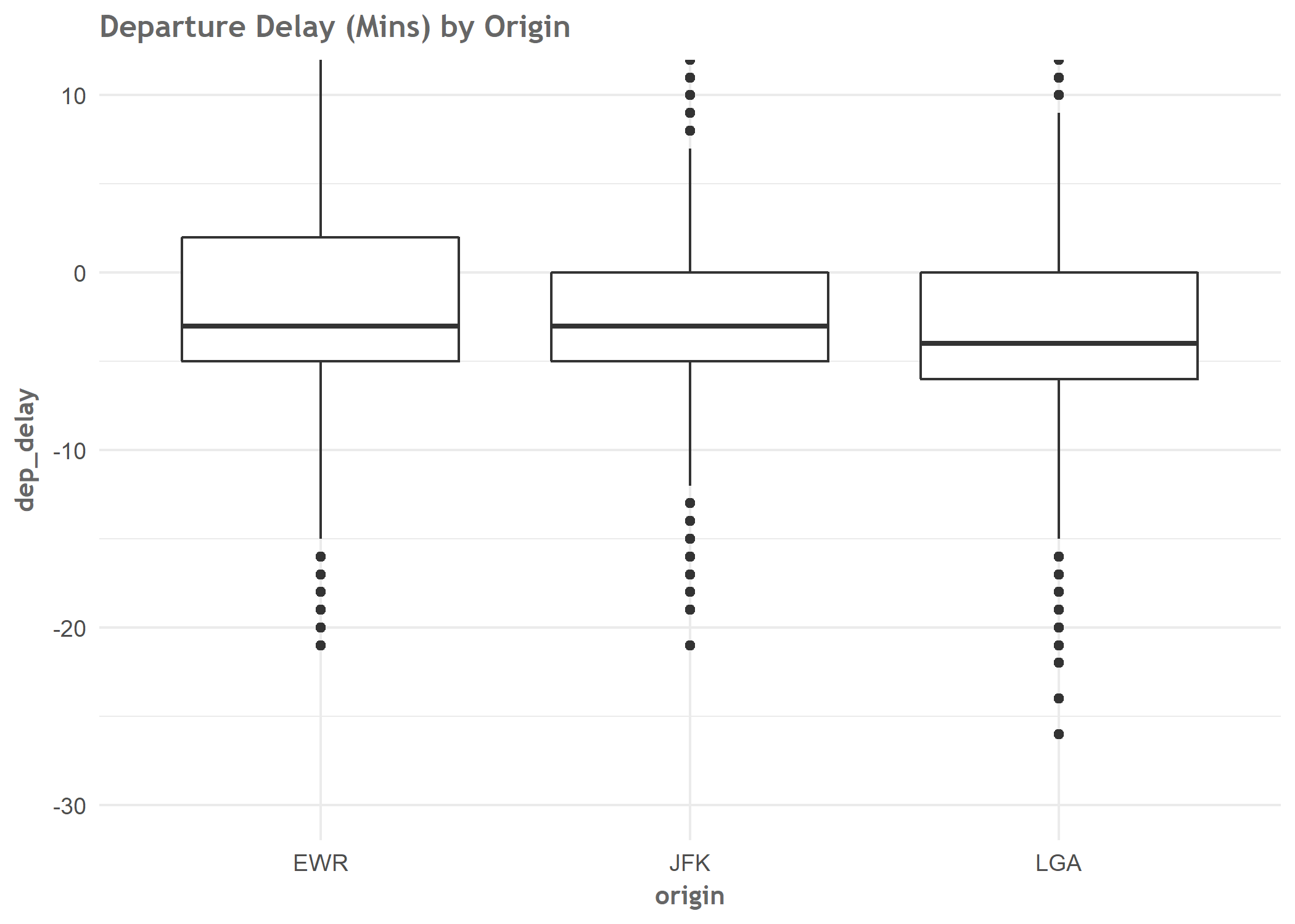ggplot(data,aes(dep_delay)) +
geom_histogram(binwidth = 1,fill = cool_blue, colour = "grey70") +
geom_vline(xintercept = 0, colour ="red") +
coord_cartesian(xlim = c(-30,10)) +
facet_wrap( ~ origin, ncol = 3) +
theme_highlandhist +
ggtitle("Departure Delay (Mins) by Origin")
ggsave("2018-03-30-DepDelayOriginHisto.png")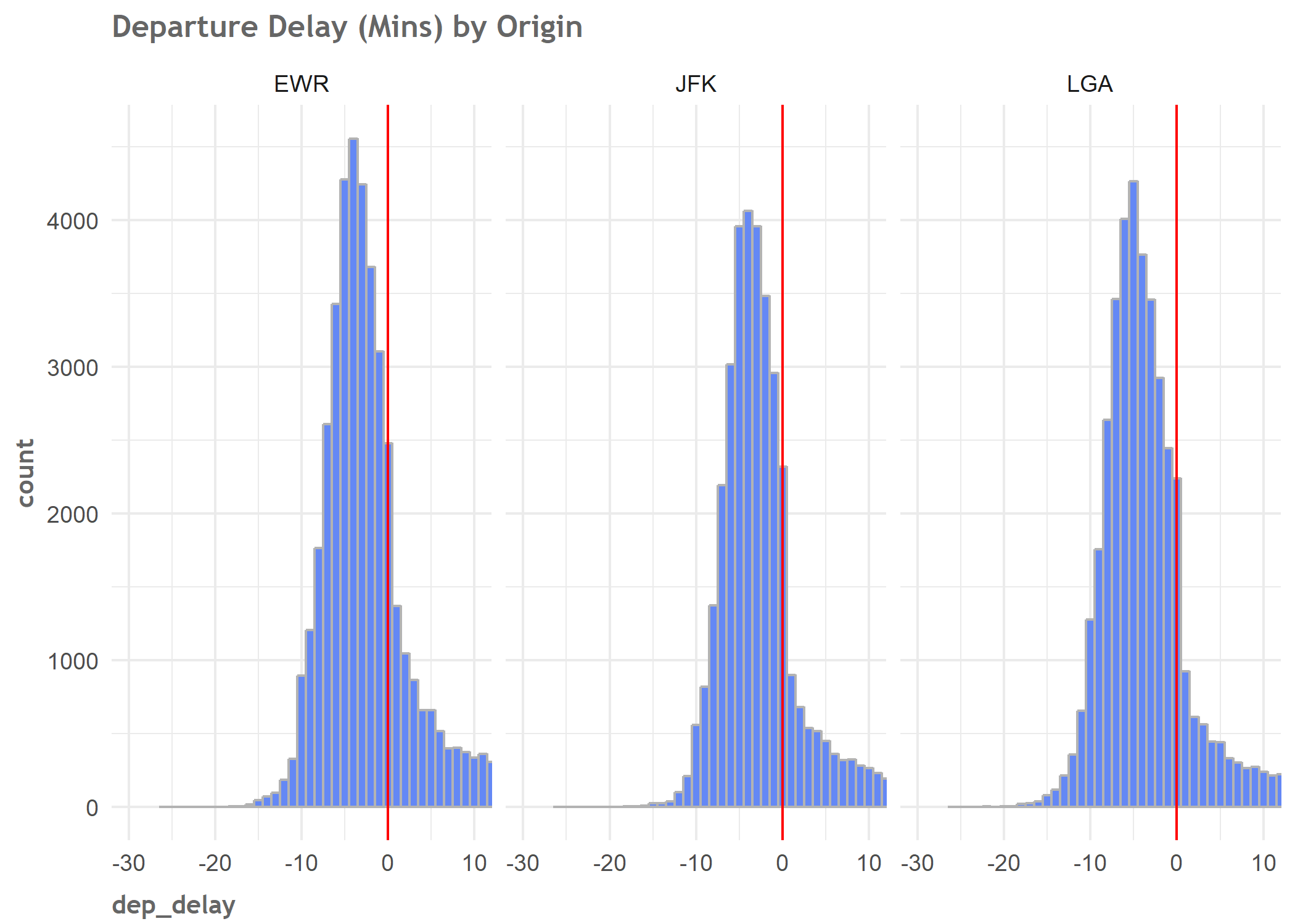We see that the median delay is less than 0 across all 3 sites. LGA has the lowest, but also has some outliers with delays greater than 10 minutes. Now let’s repeat the same plots by carrier:

#by carrier
ggplot(data,aes(carrier,dep_delay)) +
geom_boxplot() +
theme_highlandr +
coord_cartesian(ylim = c(-30,10)) +
ggtitle("Departure Delay (Mins) by Carrier")
ggsave("2018-03-30-DepDelayCarrierBoxplot.png")

ggplot(data,aes(dep_delay)) +
geom_histogram(binwidth = 1,fill = cool_blue, colour = "grey70") +
coord_cartesian(xlim = c(-30,10)) +
geom_vline(xintercept = 0, colour ="red") +
facet_wrap( ~ carrier) +
theme_highlandhist +
ggtitle("Departure Delay (Mins) by Carrier")
ggsave("2018-03-30-DepDelayCarrierHisto.png")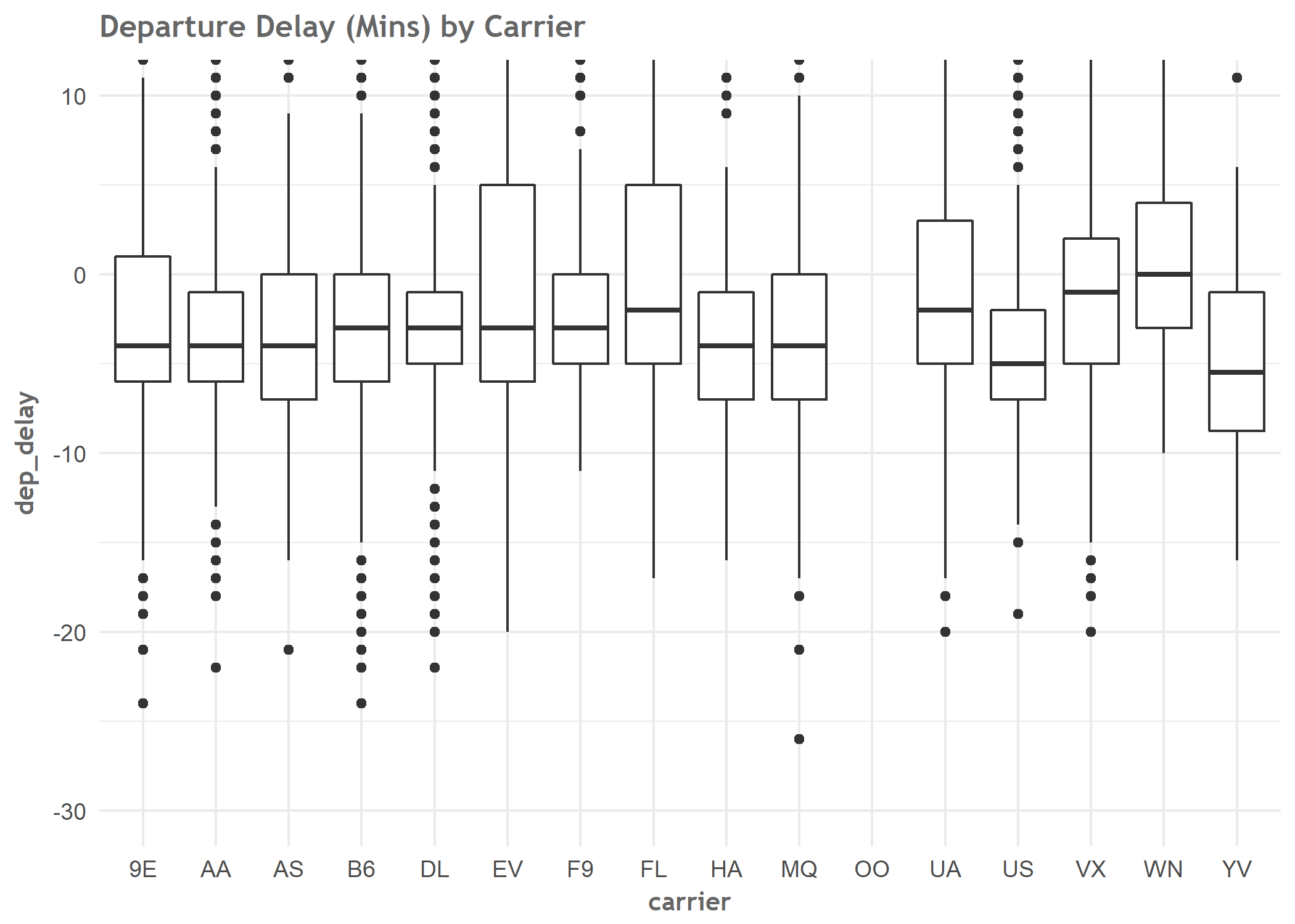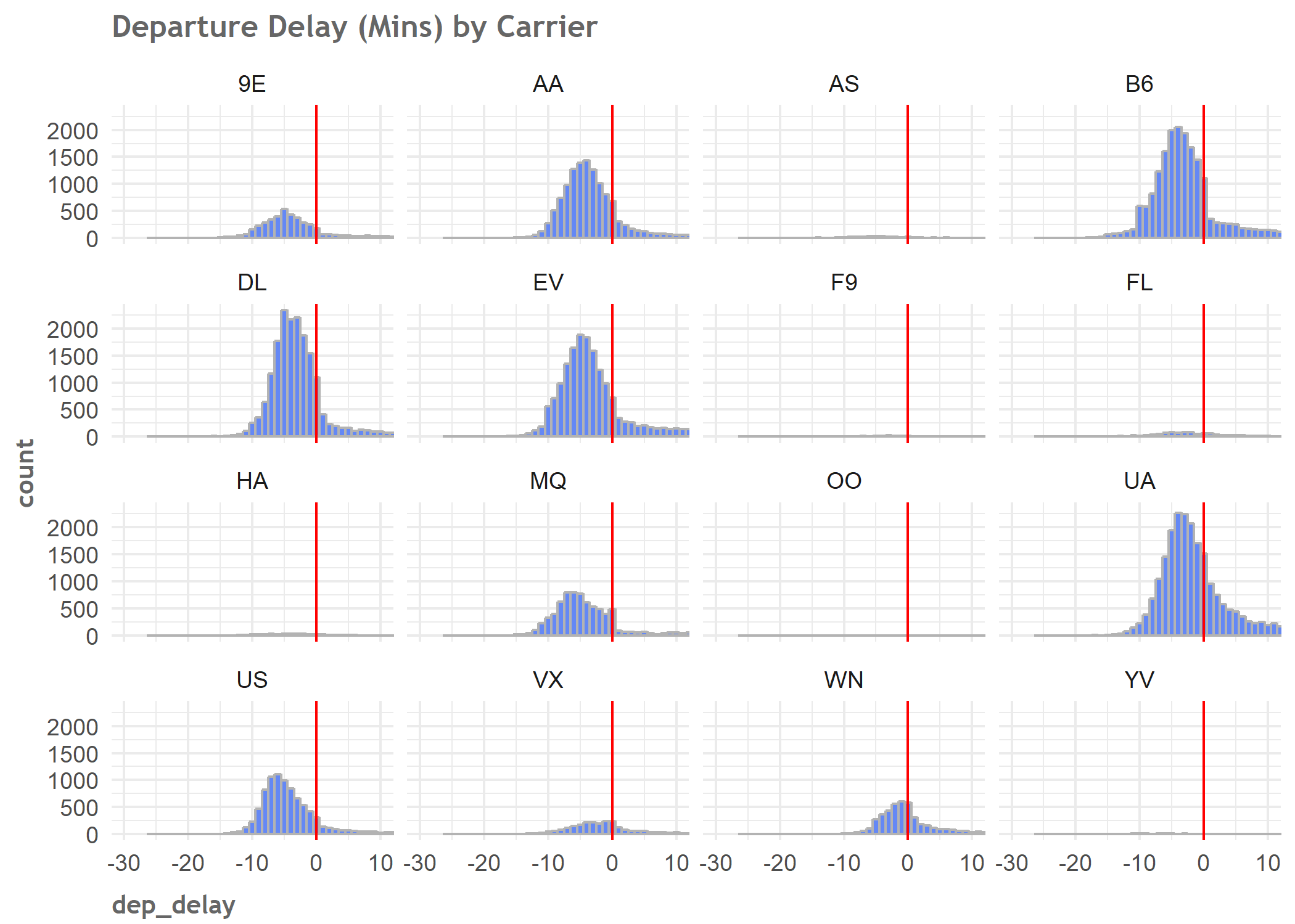For the rest of this project let’s remove the rows where the carrier is “OO” -there aren’t many - and then plot the flights by origin and carrier by month.

The boxplots and histograms give us a general idea of the data but we don’t know if this performance has been steady for a long period, or whether the observations that are the wrong (right hand) side of 0 on the histograms, or the outliers on the boxplots, are a recent phenomenon.

It’s always a good idea to plot data over time. That’s the really hot tip from this blog post!

#remove OO
data_filtered <- data %>% filter(carrier != 'OO')

monthcnt <- data_filtered %>% group_by(origin,carrier,month) %>% count()

ggplot(monthcnt,aes(factor(month),n)) +
geom_line(group = 1 ) +
geom_point(group = 1) +
theme_highlandr +
facet_wrap(origin ~ carrier, scales = "free_y") +
ggtitle("Monthly Flights by Origin ~ Carrier")
ggsave("2018-03-30-OriginCarrierOverTime.png")

table(data_filtered$origin,data_filtered$carrier)

data_filtered$origcar <- paste0(data_filtered$origin,data_filtered$carrier)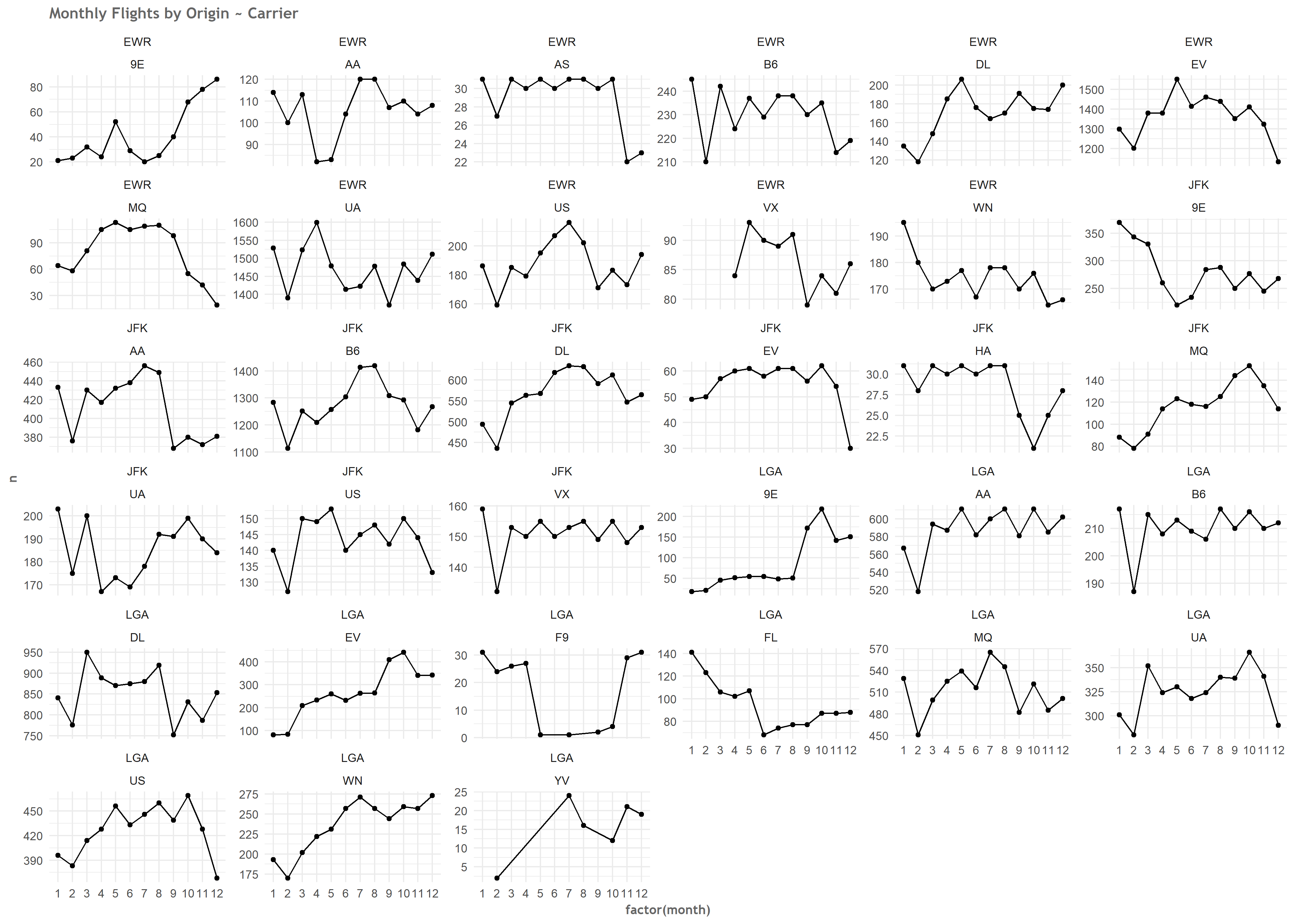Now we’ll start to use the qicharts2 package. Firstly, we’ll plot some run charts, to see if there are any signals. The run chart looks to see how often plotted points cross the centre line (for a run chart, this is a median, rather than an average). There are various run chart rules, and the package author has implemented his own additional rules, based on the number of theoretical crossings (points crossing the centre line) that should occur for a given number of points. If none of this makes sense, I encourage you to read the package vignettes, which explain all the chart types and the rules. This is hands down one of the best examples of a package vignette I have seen to date. # run chart by month monthly_rc <- qic(month, dep_delay, data = data_filtered, facets = ~ origcar, chart = 'run', title = 'Average departure delay (run chart)', ylab = 'avg dep delay', xlab = 'Month') monthly_rc ggsave("2018-03-30-monthlyrunchart.png")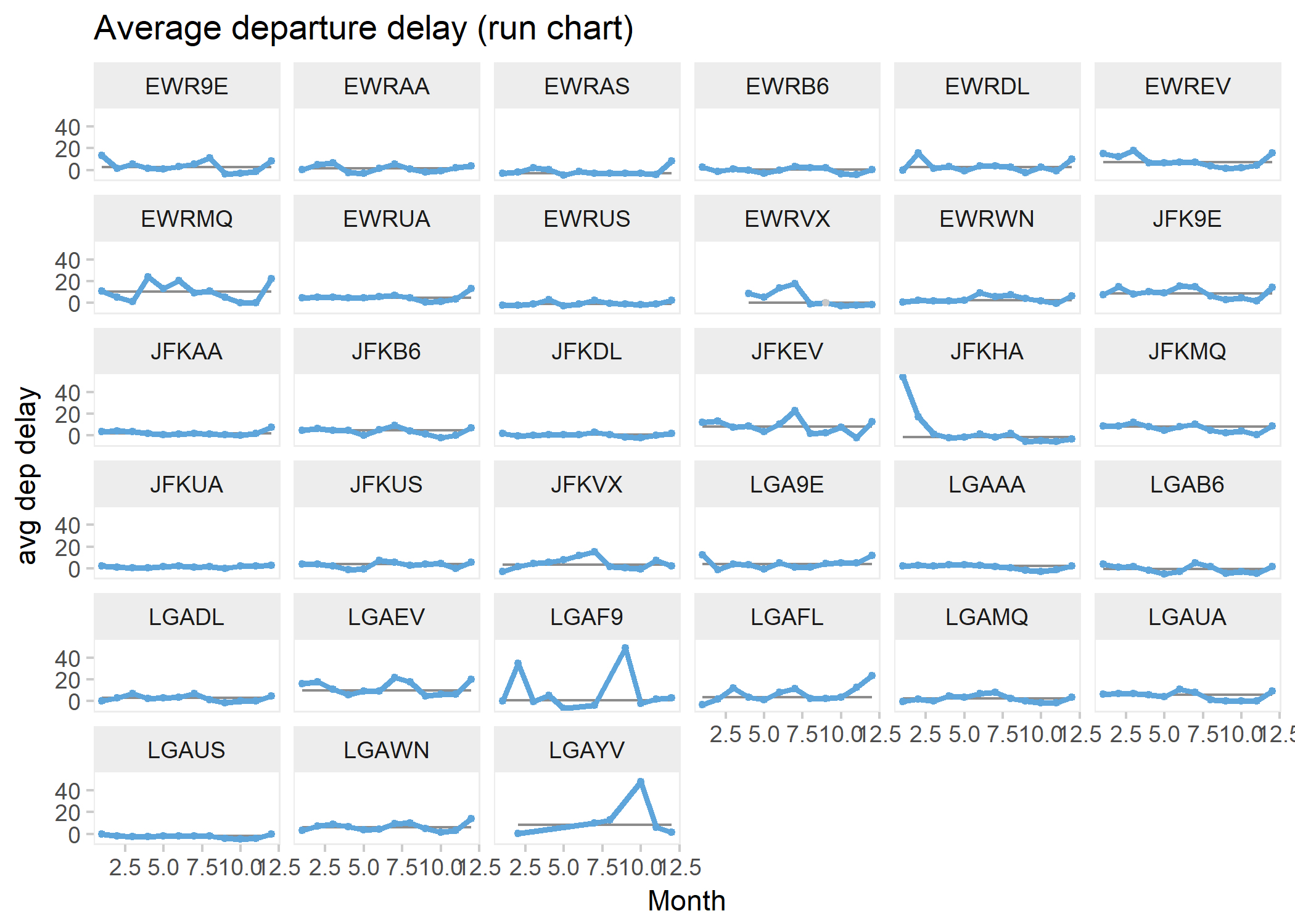We don’t see to many (or indeed, any) signals here so we can try our hand at plotting some Statistical Process Control (SPC) charts. Again, these look for certain patterns in the data relating to the centre line (for SPC charts, the centre line is usually an average). There are various types of SPC chart, dependent on the nature of your data (e.g. counts, percentages etc.) For this example we can use the “xbar” chart, as this chart allows us to have multiple measurements per subgroup ( we’re averaging all the departure delays by month). Incidentally, this is not a chart I’ve ever had to use in real life, so its good to get a chance to use it here. p <- qic(month, dep_delay, data = data_filtered, facets = ~ origcar, chart = "xbar") p ggsave("2018-03-30-qicxbar.png")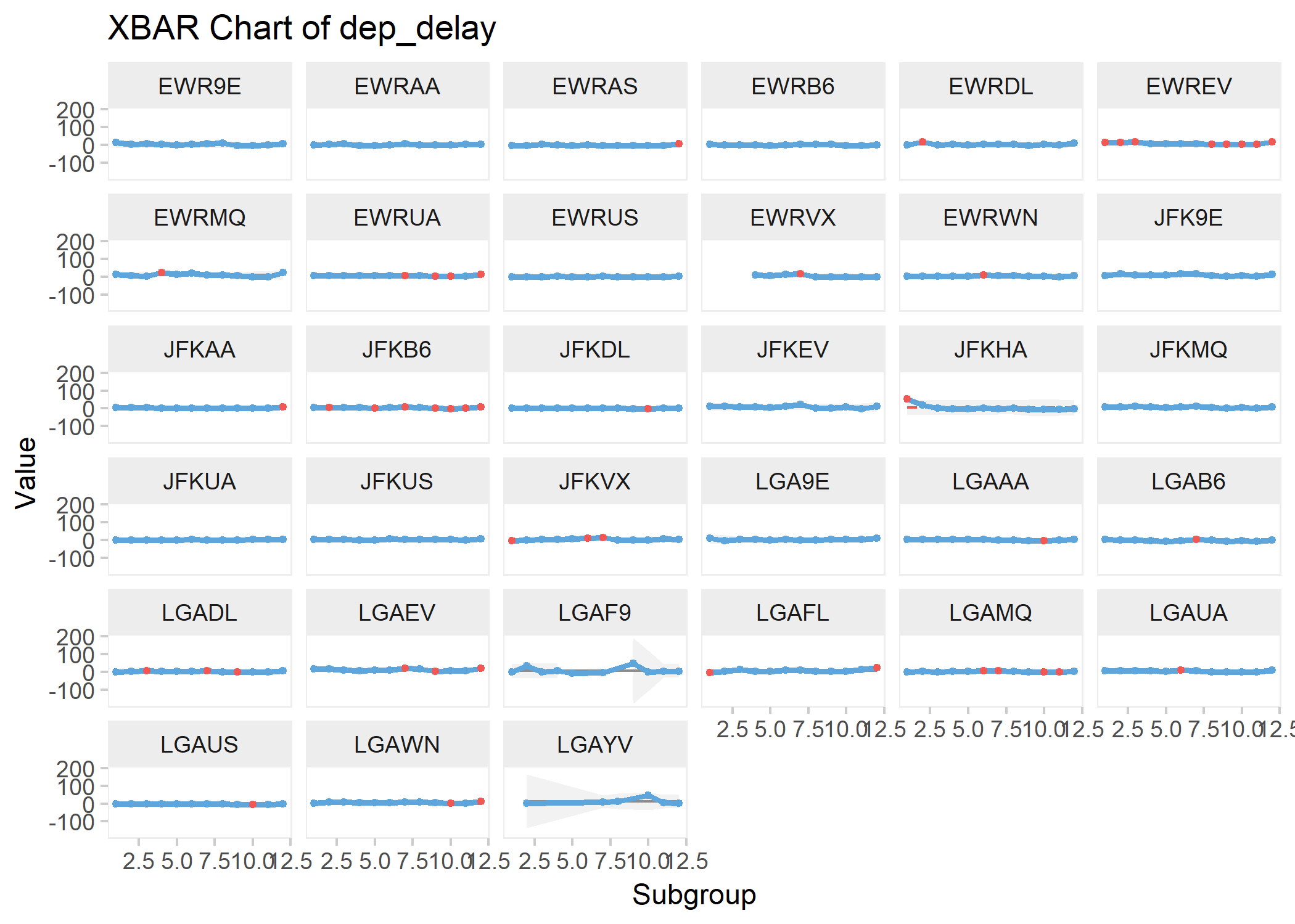The qicharts2 package is really useful, I use it a lot but I quite often want to tweak the graphs. I’ve seen a few questions on Stack Overflow from people looking to amend the graphical outputs. In fact, these are the only questions I have felt sufficiently brave enough to provide answers for on Stack Overflow. The charts are ggplot2 objects, which may not be apparent, so you can amend them by tagging on ggplot2 syntax, or you can take the data generated by the qic function, and do your own thing. This is the approach we’ll use here. First we’re going to grab the data from the qic function. Then we’re going to generate a small dataframe of all the points outside of the calculated control limits. Finally, we’ll use the data that the qic function has generated but we’ll create our own plot from the ground up using regular ggplot2 syntax. One particular change being made here is setting the background limits to a darker colour. I find the default colour doesn’t render particularly well on my laptop display.  p <- p$data

# get data beyond limits
outofcontrol <- select(p,x,y,facet1,sigma.signal) %>%
group_by(facet1) %>%
filter(sigma.signal)

xbarchart <- ggplot(p,aes(x,y)) +
geom_ribbon(ymin = p$lcl,ymax = p$ucl, fill = "grey20",alpha = 0.4) +
geom_line(colour = cool_blue, size = .75) +
geom_line(aes(x,cl)) +
geom_point(colour = cool_blue, fill = cool_blue, size = 1.5) +
geom_point(data = outofcontrol,aes(x,y),colour = "red", fill = "red",size = 1.75) +
facet_wrap(~ facet1) +
theme_highlandr +
ggExtra::removeGridX() +
ggExtra::rotateTextX() +
ggtitle(label = "Average departure delay (Xbar chart)",
subtitle = "By Carrier and Origin") +
labs(x = "Month",
y = "Avg Delay (mins)",
caption = "source: nycflights13")
xbarchart
ggsave("2018-03-30-xbar.png")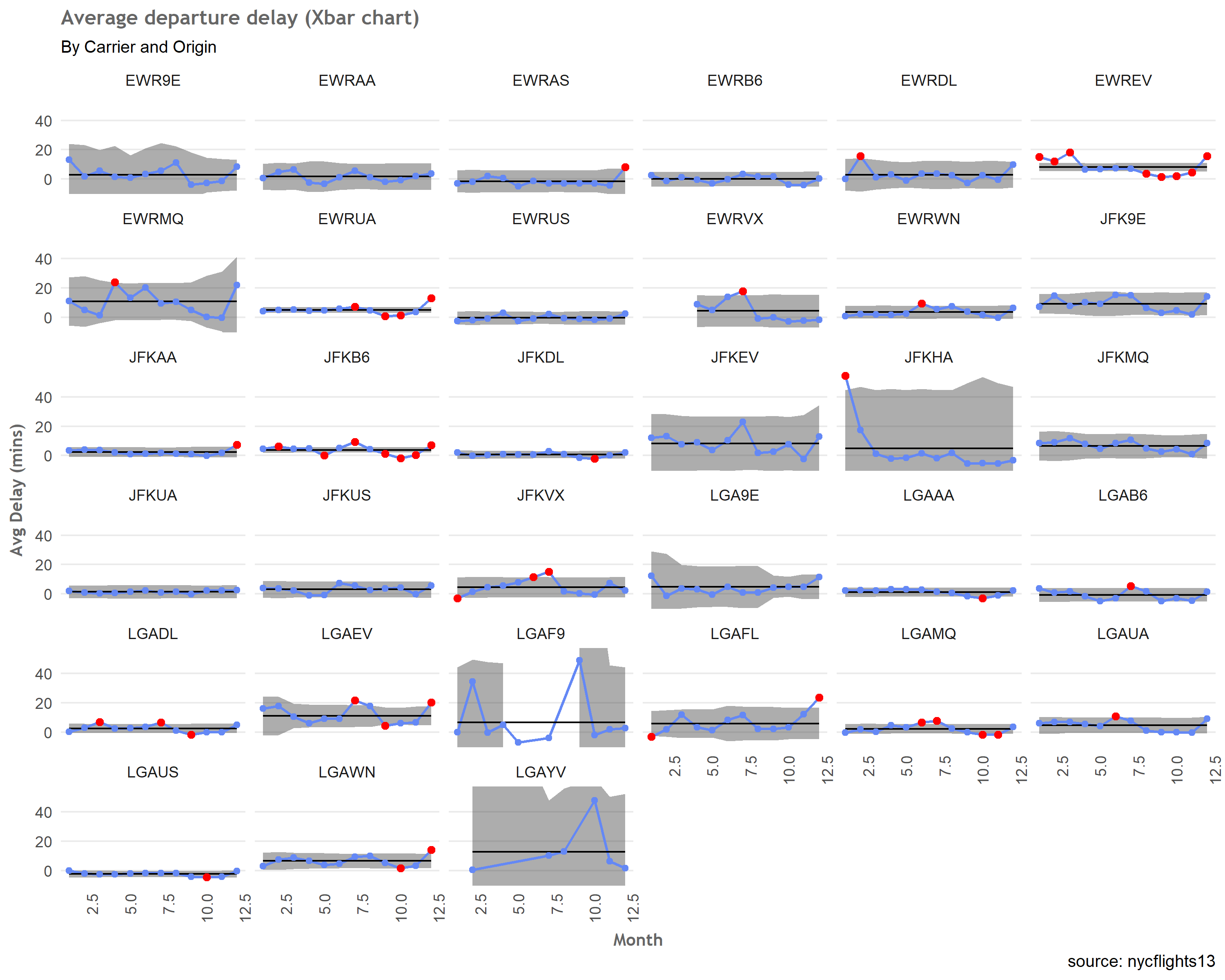It’s fairly easy to see the limits now, denoted by the darker background, with the points outside the limits in red. The wider the control limits, the more variation there is in average departure delay. Ideally we want to find the combination of Origin ~ Carrier that has very narrow limits,and an upper control limit as close to 0 as possible (meaning they don’t usually have delays).

We can start to drill down a bit using coord_cartesian to narrow our axis limits. Always use coord_cartesian when you want to zoom in on your data - it doesn’t discard points outside of the limits you set.
For example,if you are creating a boxplot, and want to zoom in, the values relating to the median,lower and upper quartiles etc will not change if you use coord-cartesian. If however, you set new limits manually, then you are effectively throwing away the previous points that now lie outside those limits adnd the boxplot will adjust accordingly.

Here I’m looking at limits between -10 (Lower Control Limit) and 10 (Upper Control Limit), and then, do we have any combinations where the points all lie between a lower end of -10 (LCL) and an upper limit of 0 (UCL)?

closeup <- xbarchart +
coord_cartesian(ylim = c(-10,10))
closeup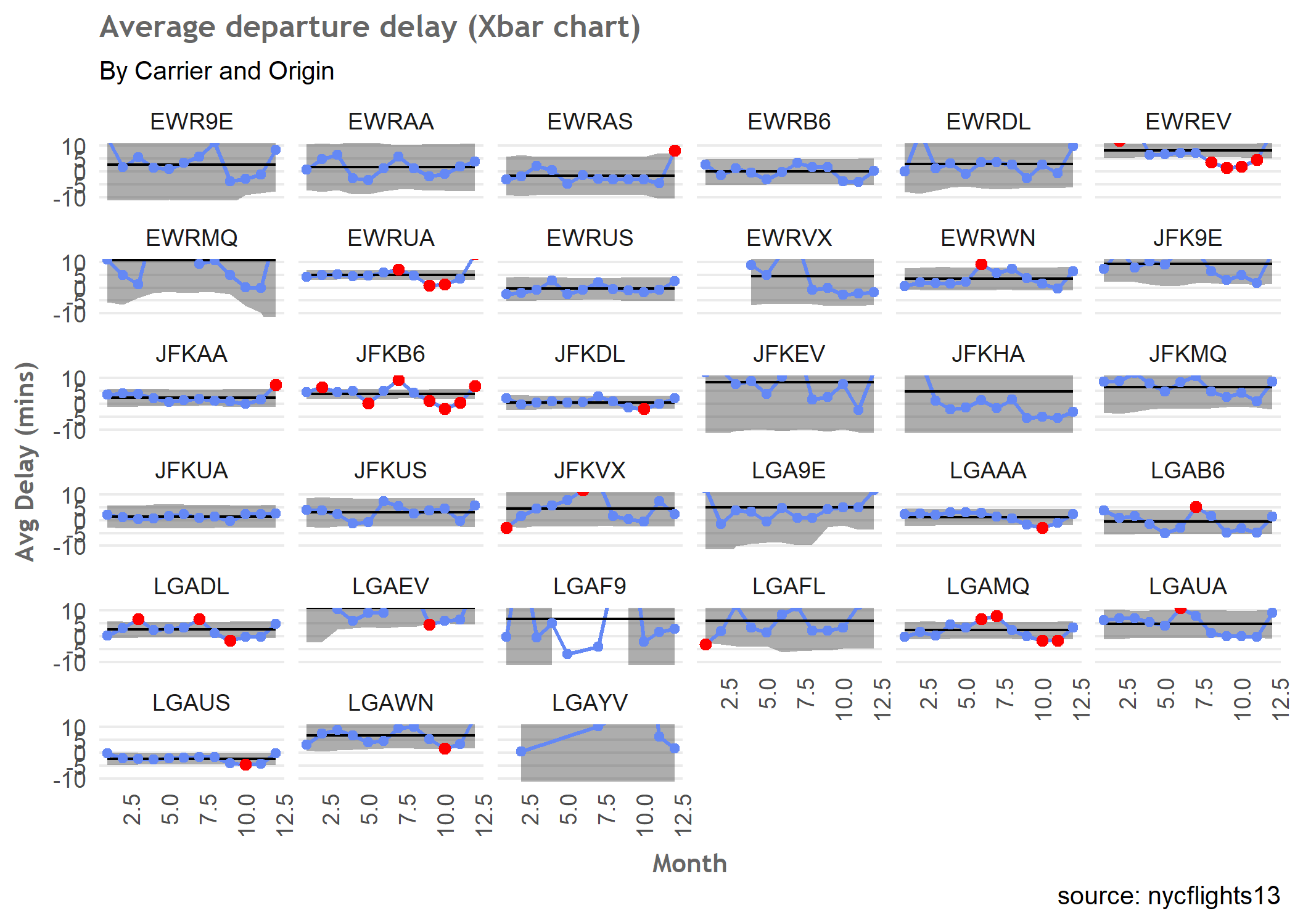revised_closeup <- xbarchart +
coord_cartesian(ylim = c(-10,0))
revised_closeup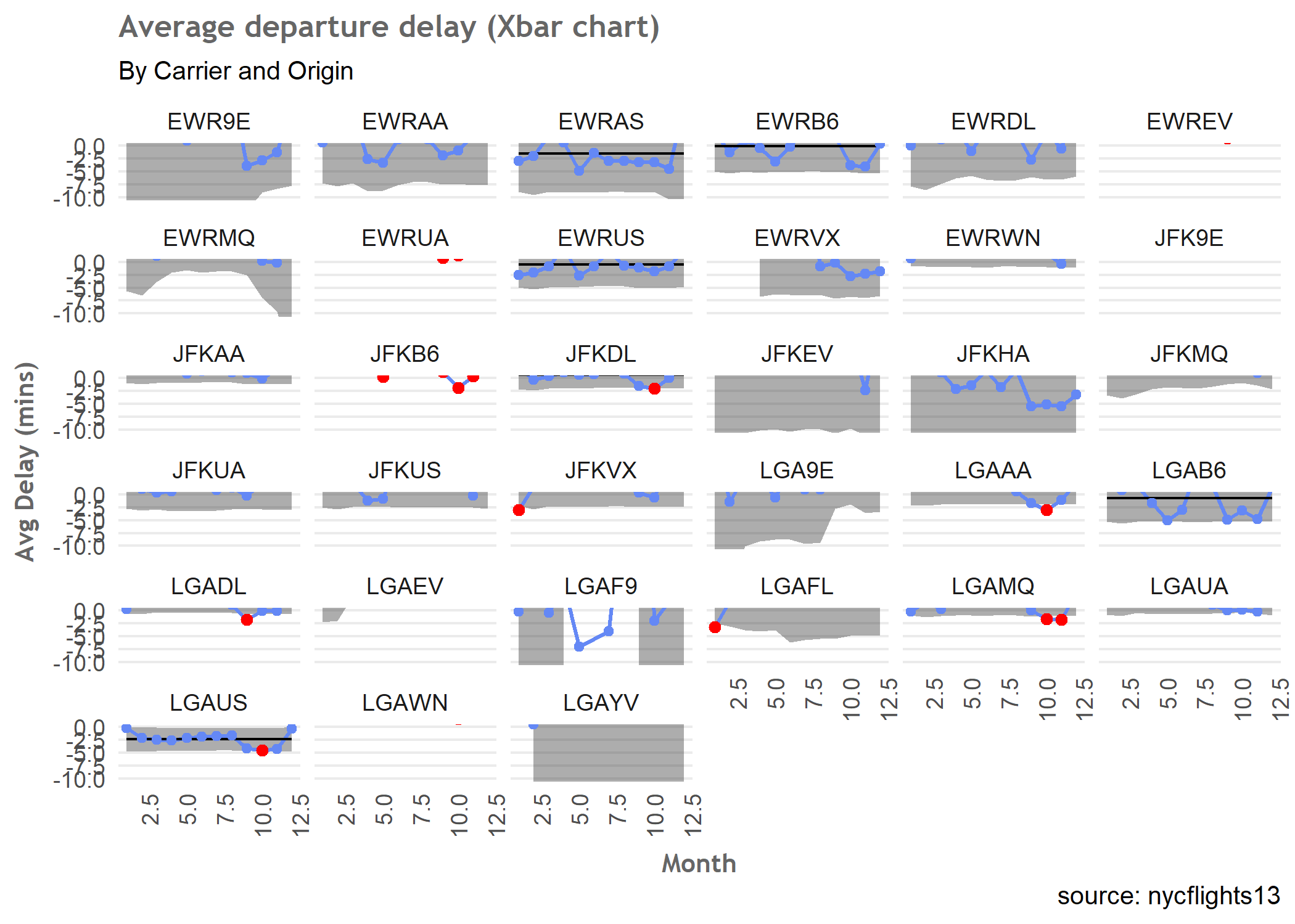OK - let’s try and get the top 2 combinations per Origin so that we can answer the question

newdata <- p

top_six <- newdata %>%
mutate(origin = stringr::str_sub(facet1,1,3)) %>%
group_by(origin) %>%
arrange(cl) %>%
distinct(facet1,cl) %>%
top_n(-2,cl) %>%
ungroup()

knitr::kable(top_six)


I want to plot these but before I do, I need to do some manipulation of the limits so that I can superimpose them on the final chart. A little bit of rounding, plus some conversion to mm:ss format :

top6 <- newdata %>%
filter(facet1 %in% c('LGAUS','EWRAS','LGAB6','EWRUS','JFKDL','JFKUA'))

top6_out_of_control <- outofcontrol %>%
filter(facet1 %in% c('LGAUS','EWRAS','LGAB6','EWRUS','JFKDL','JFKUA'))

top6$cl.lab <- round(top6$cl.lab,2)
top6$abs_cl <- abs(top6$cl.lab)
top6$abs_cl <- round(top6$abs_cl,2)

top6$cl_ms <- ifelse(!is.na(top6$abs_cl),
paste(top6$abs_cl %/% 1, stringr::str_pad(60 * (top6$abs_cl - (top6$abs_cl %/% 1)), width = 2, pad = 0), sep = ":"), NA)  Finally, we can plot our top 6 performers in terms of minimal departure delays: xbarchart <- ggplot(top6,aes(x,y)) + geom_ribbon(ymin = top6$lcl,ymax = top6\$ucl, fill = "grey20",alpha = 0.4) +
geom_line(colour = cool_blue, size = .75) +
geom_line(aes(x,cl)) +
geom_point(colour = cool_blue, fill = cool_blue, size = 1.5) +
geom_point(data = top6_out_of_control,aes(x,y),colour = "red", fill = "red",size = 1.75) +
facet_wrap(~ facet1,ncol = 2) +
theme_highlandr +
ggExtra::removeGridX() +
ggExtra::rotateTextX() +
ggExtra::removeGrid() +
ggtitle(label = "Average departure delay (Mins)",
subtitle = "By Carrier and Origin") +
labs(x = "Month",
y = "Avg Delay (mins)",
caption = "source: nycflights13") +
geom_text(aes(label = cl_ms,x = 11, y = cl + 1.2),na.rm = TRUE)
xbarchart

ggsave("2018-03-30-finalplot.png")So there you have it. These appear to be the best 2 combo’s for each origin in order to minimise the chance of running into a delayed departure. I’d be interested to see how others would tackle this, and what alternative answers might be?